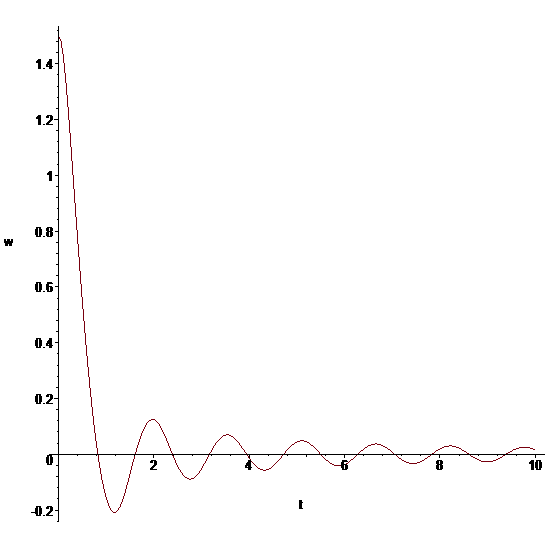# Motion of a non-linear pendulum with air resistance

## Homework Statement

I need to come up with an equation that would model the motion of a non-linear pendulum with air resistance. [/B]

## Homework Equations

Fc=mgsintheta
Fdrag=(1/2)p(v^2)CA

## The Attempt at a Solution

I started with mgsintheta-(1/2)p(v^2)CA=ma

After substituting v=r*omega and a=r*alpha, I get the following (look at the image)However, after graphing, I do not get a damped oscillating curve, because of the v^2 not changing signs. Can someone guide me? See second image:http://prntscr.com/i6bh0j[/B]

https://prnt.sc/i6bfv0

Sorry, we can't see the 1st image

epenguin
Homework Helper
Gold Member
To know whether there is a problem, how many cycles did you follow it through?

To know whether there is a problem, how many cycles did you follow it through?
I'm not quite sure what you mean by cycles. I set the domain on the graph to be [0, 2pi].

epenguin
Homework Helper
Gold Member
I'm not quite sure what you mean by cycles. I set the domain on the graph to be [0, 2pi].
To be sure there is no damping try 20π or 100π?

It does make sense that your damping doesn't work if the damping force is always pointing in the same direction and not against v. Maybe you should try to replace v^2 by |v|v.

•Douglas Sunday
To be sure there is no damping try 20π or 100π?
I did, and there does not seem to be any. However, the amplitude does seem to change, but on average it remains the same.

It does make sense that your damping doesn't work if the damping force is always pointing in the same direction and not against v. Maybe you should try to replace v^2 by |v|v.
Thanks, this does work. For some reason I didn't think of the absolute value as a solution to the v^2 problem. Now I get a graph that looks like the following. It does not pass through the x-axis like it should.
https://prnt.sc/i6cgpx

Thanks, this does work. For some reason I didn't think of the absolute value as a solution to the v^2 problem. Now I get a graph that looks like the following. It does not pass through the x-axis like it should.
https://prnt.sc/i6cgpx

Another sign error. When you're at positive theta, the force must point in the negative direction. So it should be -mgsin(theta).

Another sign error. When you're at positive theta, the force must point in the negative direction. So it should be -mgsin(theta).
yes, I thought I already changed that. Thanks again.

Another sign error. When you're at positive theta, the force must point in the negative direction. So it should be -mgsin(theta).
Did you look at the derivation of that equation I came up with? I just need someone to check if its correct (apart from the signs, and absolute values)

Did you look at the derivation of that equation I came up with? I just need someone to check if its correct (apart from the signs, and absolute values)

Seems fine to me.

Ray Vickson
Homework Helper
Dearly Missed
Seems fine to me.

Here is what I get using Maple to solve the IVP
$$\theta^{''} = -\frac{g}{L} \sin(\theta) - \frac{pLcA}{2m} \theta' |\theta'|, \; \theta(0)=1.5, \theta'(0)=0$$#### Attachments

Last edited:
Mark44
Mentor
Thread moved. Problems involving differential equations belong in the Calculus & Beyond section.